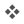##Time Value Of Money - Financial Calculator

Our online Time Value of Money calculator allows you to learn the concepts and calculate the Present Value, Monthly Payment, Future Value, Interest Rate and Time Period for a loan, mortgage or lease.

Simple TVM Calculator

Set four of the five values. Click a button for the value you want to find.

Interest Rate
%
Term
Years
Present Value
Payment
Future Value

Payment Timing - Compounded Monthly

At the end of each month (e.g. loan or mortgage)

At the beginning of each month (e.g. lease)

## Time Value Of Money - Financial Calculator

### TVM Finanical Calculator TipsWorks Like A Business Calculator:  Set four of the five fields, then click the button for the value you'd like to calculate.Conventional Payments:  Monies going out (-), such as the monthly payment of a loan, should have the opposite sign of monies coming in (+), such as the amount borrowed or Present Value.Must have one positive (+) and one negative value (-):  Among the Present Value, Payment, and Future Value fields, there must be at least one cash outflow (-) and one cash inflow (+).Level Payments:  The periodic monthly payments are all the same for the entire duration.

### TVM Financial Calculator FieldsPresent Value:  The Present Value is the value at the beginning. In a car loan or mortgage, the present value is the amount borrowed at the beginning of the loan. Since the borrower is receiving the monies, the cashflow can be considered positive (+) to the borrower.Payment:  The Payment is the periodic cash inflows or outflows. This calculator is configured for monthly payments, or 12 payments per year. In a car loan or mortgage, the borrower makes monthly payments. These cash outflows can be considered negative (-) to the borrower.Future Value:  The Future Value is the value of the last cashflow at the very end of the time horizon. In a car loan or mortgage, if the loan is to be paid completely off, the future value is zero. In a lease, the future value is the residual value in the lease.Interest Rate:  The Interest Rate field is the percentage Nominal Annual Rate. Since this financial calculator is set to monthly payments, this interest is compounded monthly. For this calculator, the Nominal Annual Rate is divided by 12 to determine the Periodic Rate.Years:  The Years field is the total time horizon from time zero of the first cashflow until the last cashflow which is the future value. The time horizon encompasses all the periodic monthly payments

.